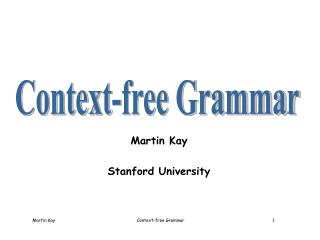DownloadDownload PresentationMartin Kay Stanford University

# Martin Kay Stanford University

Download Presentation## Martin Kay Stanford University

- - - - - - - - - - - - - - - - - - - - - - - - - - - E N D - - - - - - - - - - - - - - - - - - - - - - - - - - -
##### Presentation Transcript

1. Martin Kay Stanford University Context-free Grammar

2. Iteration Embedding The Chomsky Hierarchy Finite Regular Context-free

3. sum prod ad_op sum base + prod ad_op base ( sum ) base - y prod ad_op sum x base + prod a base b Aithmetic Expressions sum  prod add_op sum sum  prod prod  base mul_op prod prod  base base  a | b | c ... base  ( sum ) ad_op  + ad_op  - mul_op  * mul_op  /

4. Aithmetic Expressions sum prod base ( sum ) prod base ( sum ) . . sum  prod add_op sum sum  prod prod  base mul_op prod prod  base base  a | b | c ... base  ( sum ) ad_op  + ad_op  - mul_op  * mul_op  /

5. Embedding Arbitrarily embedded parentheses are not possible in a regular language because the number of open parentheses that still requiring matching close parentheses can be greater than the number of states in the automaton, whatever that number is. Hence, by the pumping lemma, an automaton with only a finite number of states cannot characterize the language.

6. English S S NP VP NP Vd NP NP S S NP VP VP NP VP Vi Vt NP Vi John told me that it rained That it rained surprised John

7. That it rained slept soundly. Mary told the boy Mary John told that it rained me That it rained were certainly true. His arguments convinces me. Me told Mary it. Lots of Problems! For the moment, just forget the problems

8. 2n-2 Exponential How many (binary) treeswith n terminals? w w w w w w w w w w w w w 1 0 0 1 1 0 1 0 0 1 1

9. 2n - 1 n 2n - 1 Cat(n) = Catalan Numbers Terminals Trees 1 1 2 1 3 2 (= 1*1 + 1*1) 4 5 (= 1*2 + 1*1 + 2*1) 5 14 (= 1*5 + 1*2 + 2*1 + 5*1) 6 42 (= 1*14 + 1*5 + 2*2 + 5*1 + 14*1) ... Why?

10. Catalan Numbers The number of binary trees with n terminals The number of arbitrarily branching trees with n nodes. Any tree can be encoded in a binary tree with the same number of terminals as the original tree has nodes.

11. Let there be: a arcs, n nonterminals, and t terminals There are two arcs downward from each nonterminal, so a = 2n There is one arc upward from each terminal and nonterminal except the root, so a = n + t -1 Ergo n = t - 1 Catalan Numbers There are n nonterminals in the binary tree for the n nodes in the original tree, except for the root. There are n+1 terminals in a binary tree with n nonterminals. 

12. Parsing Parse(Goals, StringIn, StringOut) parse([], String, String). parse([G | Goals], [G | String], String). parse(Goals, String0, String) :- rule(G, Rhs), parse(Rhs, String0, String1), parse(Goals, [G | String1], String).